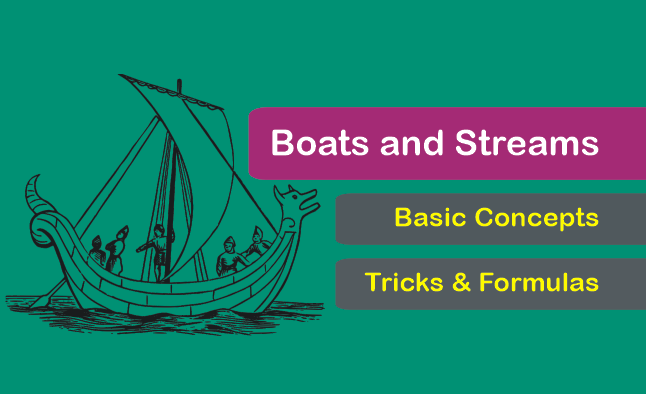# Boats and Streams: Formulas Concepts and Questions for CAT Exam

Boats and Streams: Formulas Concepts and Questions for CAT Exam
4.9 (97.58%) 33 votes

Boats and Streams is an essential topic for many competitive exams. Many varieties of questions can be framed from this area. We use the fundamental concepts of time speed and distance only to solve elementary questions on Boats and Streams.

However, some types of questions are tricky and take lots of time to solve by applying textbook approach. Fortunately, there are some shortcut formulas available to handle such problems.

In this article, besides an understanding of the basic concepts of boat sand streams, we will also learn few tricks to solve some exceptional questions.

### Boats and Streams – Terminologies: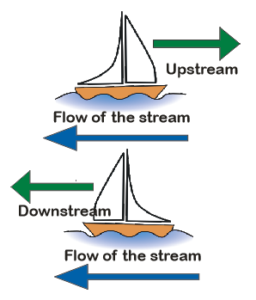Stream: It implies that the water in the river is moving or flowing.

Downstream or with the stream: It indicates that the stream favors the man’s rowing (or boating). i.e., the direction of rowing and direction of flow (stream) is same.

Upstream or against the stream: It indicates that the stream flows against the man’s rowing (or boating), i.e., the direction of rowing and direction of the stream (current) are opposite.

Some shortcut formulas related to the speed of boats and streams is handy as we require them frequently. Below are the lists of the formulas:

Let the speed of a boat (or man) in still water be X m/sec, and the speed of the stream (or current) be Y m/sec.

• Speed of boat with the stream (or Downstream or D/S) = (X + Y) m/sec.
• Speed of boat against the stream (or Upstream or D/S) = (X – Y) m/sec.
• Speed of man/boat in still water =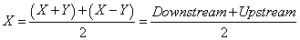• Speed of the stream =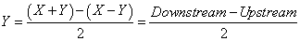### Elementary solved questions on boats and streams:

Question 1: A boat is rowed down a river 28 km in 4 hours and up a river 12 km in 6 hours. Find the speed of the boat and the river.

Explanation:

Downstream Speed = 28/4 = 7 kmph.

Upstream Speed = 12/6 = 2 kmph.

Now, applying the above formulas:

Therefore, Speed of boat in still water=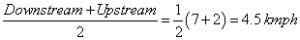Also, Speed of current/stream =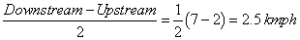Question 2: A man rows 18 km down a river in 4 hours with the stream and returns in 12 hours. Find his speed and also the speed of the stream.

Explanation:

Speed with the stream (Downstream)= 18/4 = 4.5 kmph.

Speed against the stream (Upstream) = 18/12 = 1.5 kmph.

Therefore, speed of the stream = 1/2(4.5 – 1.5) = 1.5 kmph and speed of the man = 4.5 – 1.5 = 3 kmph.

## Man/Boat moving same distance upstream and downstream

### Finding average speed if moving with different upstream and downstream speeds

Important: If a man capable of rowing at the speed of x m/sec in still water, rows the same distance up and down a stream flowing at a rate of y m/sec, then his average speed throughout the journey is =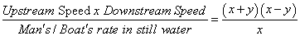Question 33: The speed of the boat in the still water is 6 kpmh, and the speed of the stream is 2 kmph. The boat goes a certain distance down the stream and comes back upstream to the same place from where it started. In the journey, the boat didn’t stop at any point. Find the average speed of the boat in the entire journey.

Standard Method:

Let one side the distance be d km.

Downstream speed = 6+2 = 8 kmph

Similarly, Upstream speed = 6-2 = 4 kmph.

Therefore, the average speed of the boat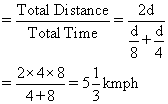Shortcut Formula:

Average Speed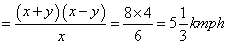### Finding the ratio of speeds of the boat and the stream

Important: If the boat takes tdown and tup respectively to travel same downstream and upstream distance, then we the following relation: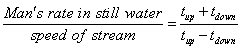Question 4: A boat takes 6 hours to go downstream and comes back upstream in 12 hours. Find the ratio of the speed of the boat and the speed of the stream.

Standard Method:

Let the common distance be d km.

Hence, Downstream speed = x+y and upstream speed = x-y.

Therefore, time taken to cover downstream distance = d/(x+y)=6 … (1)

Similarly, time taken to cover upstream distance =d/(x-y)=12 …. (2)

Dividing (2) by (1) we get,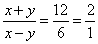Now, applying componendo and dividendo in the above equation, we get: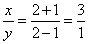Hence, the required ratio is 3:1.

Shortcut Formula:
On comparing, tdown = 6 and tup =12

Therefore,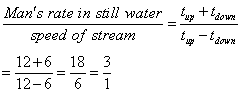Or, the required ratio = 3:1.

### Finding the total time of the journey.

Important: If a boat has a speed of x m/sec in still water, rows the same distance (say d km) up and down and the stream flowing at a rate of y m/sec, then the total time taken for the journey is: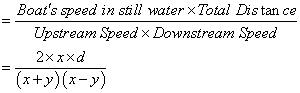Question 5: A man rows 10 km upstream and back again to the starting point in 55 min. If the speed of the stream is 2 kmph, then find the speed of the boat in still water.

Shortcut Formula:

Let the speed of the boat in still water be x kmph. Now, applying the formula, we have: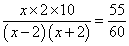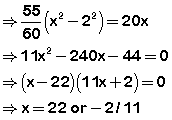Discarding the negative value, the speed of the boat in still water = 22 kmph.

## Time Speed and Distance